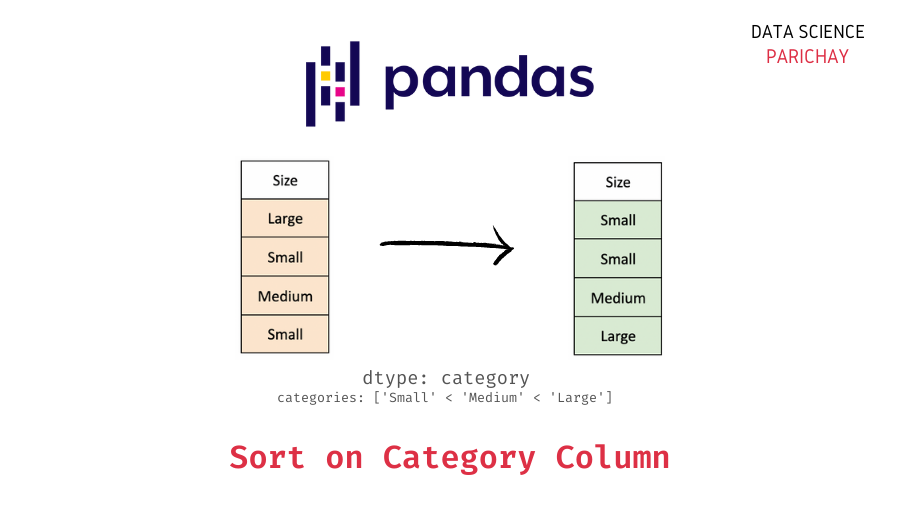# Pandas – Sort Dataframe on Category Column

In this tutorial, we will look at how to sort a Pandas dataframe based on values in a category type column with the help of some examples.

## How to sort a dataframe on a category column in Pandas?

You can use the Pandas dataframe `sort_values()` function to sort a dataframe. Pass the category column name as an argument to the `by` parameter. This is similar to how you’d sort a dataframe on columns with other types. The following is the syntax –

```# sort dataframe by a column
df.sort_values(by="col")```

It returns the sorted dataframe.

## Examples

Let’s look at some examples of sorting a dataframe on a categorical column. First, we’ll create a sample dataframe that we will be using throughout this tutorial.

```import pandas as pd

# create a dataframe
df = pd.DataFrame({
"Name": ["Tim", "Sarah", "Hasan", "Jyoti", "Jack"],
"Gender": ["Male", "Female", "Male", "Female", "Male"],
"Shirt Size": ["Small", "Medium", "Large", "Small", "Large"]
})

# change to category dtype
df["Gender"] = df["Gender"].astype("category")
df["Shirt Size"] = df["Shirt Size"].astype("category")
# set and order categories for "Shirt Size" column
df["Shirt Size"] = df["Shirt Size"].cat.set_categories(["Small", "Medium", "Large"], ordered=True)

# display the dataframe
df```

Output:

We now have a dataframe containing the name, gender, and t-shirt size of some students in a university. Note that the “Gender” and the “Shirt Size” columns are of `category` dtype. The “Gender” column is an unordered categorical field whereas the “Shirt Size” column is an ordered categorical field.

### Sort dataframe on unordered category column

The “Gender” column in the above dataframe is an unordered category type column. Let’s print out the column.

```# display "Gender" column
print(df["Gender"])```

Output:

📚 Data Science Programs By Skill Level

Introductory

Intermediate ⭐⭐⭐

🔎 Find Data Science Programs 👨‍💻 111,889 already enrolled

Disclaimer: Data Science Parichay is reader supported. When you purchase a course through a link on this site, we may earn a small commission at no additional cost to you. Earned commissions help support this website and its team of writers.

```0      Male
1    Female
2      Male
3    Female
4      Male
Name: Gender, dtype: category
Categories (2, object): ['Female', 'Male']```

Let’s now sort the above dataframe on the “Gender” column where the category values do not have an inherent order to them.

```# sort dataframe on "Gender" column
df.sort_values(by="Gender")```

Output:

The resulting dataframe is sorted on the “Gender” column alphabetically.

### Sort dataframe on ordered category column

The categories in an ordered category column have an order to them. For example, in the “Shirt Size” column above, the category order is “Small” < “Medium” < “Large”. Let’s display this column first to see its values and the category order.

```# display the "Shirt Size" column
print(df["Shirt Size"])```

Output:

```0     Small
1    Medium
2     Large
3     Small
4     Large
Name: Shirt Size, dtype: category
Categories (3, object): ['Small' < 'Medium' < 'Large']```

Let’s now sort the above dataframe on the “Shirt Size” column.

```# sort dataframe on "Shirt Size" column
df.sort_values(by="Shirt Size")```

Output:

Note that the sorted dataframe has values sorted according to the category order. You can see that rows with “Small” in the “Shirt Size” column come first, then rows with “Medium” and finally rows with “Large” as “Shirt Size”.

The behavior of sorting a dataframe on column values is similar if you use other column types. Keep in mind that ordered category columns will be sorted according to the defined category order.

You can also perform multi-column sort in a similar way. For example, let’s sort the above dataframe on the columns “Gender” and “Shirt Size” together. For this, pass “Gender” and “Shirt Size” as a list to the `by` parameter.

```# sort dataframe on "Gender" and "Shirt Size" column
df.sort_values(by=["Gender", "Shirt Size"])```

Output:

Here, the dataframe is first sorted on the “Gender” column and then on the “Shirt Size” column (which can help sort rows having the same “Gender” value).

You might also be interested in –

•